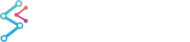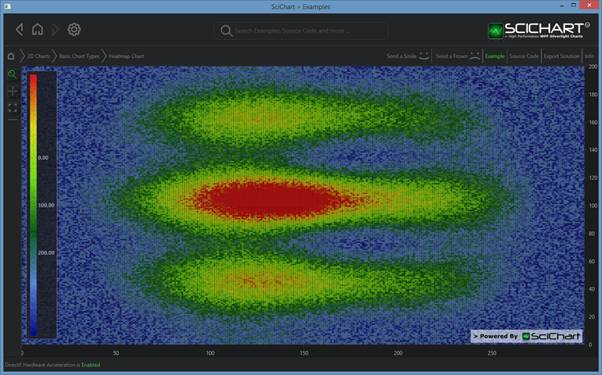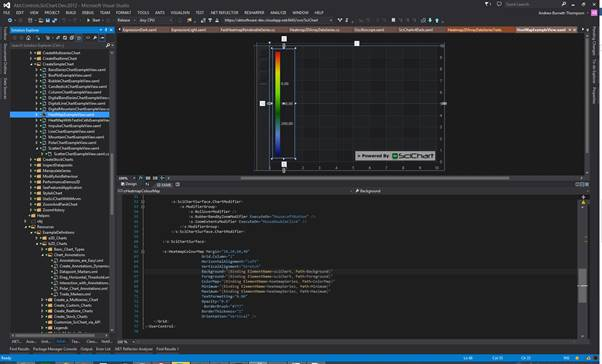﻿ The Heatmap Type | WPF Chart DocumentationSciChart WPF 2D Charts > 2D Chart Types > The Heatmap Type
The Heatmap Type

SciChart WPF v5 provides two heatmap types: the FastUniformHeatmapRenderableSeries and the FastNonUniformHeatmapRenderableSeries. These two series types are designed to display 2D arrays of data with real values. Every item in the 2D array is represented as a colored rectangle (“cell”); the color depends on corresponding item’s value.

Examples for the Heatmap Chart can be found in the SciChart WPF Examples Suite which can be downloaded from the SciChart Website or our SciChart.WPF.Examples Github Repository.NOTE: The FastheatmapRenderableSeries type has been made obsolete in v5 of SciChart. Please use the new FastUniformHeatmapRenderableSeries and FastNonUniformHeatmapRenderableSeries type.

## Uniform Heatmaps

Uniform heatmaps are extremely fast, lightweight series types for rendering two dimensional data as a heatmap or spectrogram. The FastUniformRenderableSeries type should be used in conjunction with a UniformHeatmapDataSeries when you simply want to specify a Step in the X,Y direction (each cell is the same size).

For non-uniform heatmaps, scroll down...

### Example: Declaring a Uniform Heatmap in Xaml / Code Behind

Declaring a FastHeatMapRenderableSeries
Copy Code
```<s:SciChartSurface x:Name="sciChart" Padding="0" BorderThickness="0">
<s:SciChartSurface.RenderableSeries>
<s:FastUniformHeatmapRenderableSeries x:Name="heatmapSeries" Opacity="0.9">
<s:FastUniformHeatmapRenderableSeries.ColorMap>
<s:HeatmapColorPalette Maximum="1">
</s:HeatmapColorPalette>
</s:FastUniformHeatmapRenderableSeries.ColorMap>
</s:FastUniformHeatmapRenderableSeries>
</s:SciChartSurface.RenderableSeries>
<s:SciChartSurface.XAxis>
<s:NumericAxis DrawMajorBands="True" />
</s:SciChartSurface.XAxis>
<s:SciChartSurface.YAxis>
<s:NumericAxis DrawMajorBands="True" />
</s:SciChartSurface.YAxis>
</s:SciChartSurface>

// Then, in code-behind, apply the data
int cellHeight = 200;
int cellWidth = 300;
var data = new double[cellHeight, cellWidth];
// TODO: Fill the data[,] array with values to represent your heat values
// NOTE: HeatmapColorPalette.Maximum and Minimum defines the mapping of gradient colors to data values

double startX = 0;
double stepX = 1;
double startY = 0;
double stepY = 1;
var heatmapDataSeries = new UniformHeatmapDataSeries<double, double, double>(data, startX, stepX, startY, stepY);

// Apply the dataseries to the heatmap
heatmapSeries.DataSeries = heatmapDataSeries;
```

### Example: Declaring a Uniform Heatmap in pure code

Declaring a FastHeatMapRenderableSeries
Copy Code
```// First, declare the FastUniformHeatmapRenderableSeries
var heatmapRenderableSeries = new FastUniformHeatmapRenderableSeries()
{
ColorMap = new HeatmapColorPalette()
{
Maximum = 200,
Minimum = 0,
}
};

// Then, in code, apply the data
int cellHeight = 200;
int cellWidth = 300;
var data = new double[cellHeight, cellWidth];
// TODO: Fill the data[,] array with values to represent your heat values
// NOTE: HeatmapColorPalette.Maximum and Minimum defines the mapping of gradient colors to data values

double startX = 0;
double stepX = 1;
double startY = 0;
double stepY = 1;
var heatmapDataSeries = new UniformHeatmapDataSeries<double, double, double>(data, startX, stepX, startY, stepY);
// Apply the dataseries to the heatmap
heatmapSeries.DataSeries = heatmapDataSeries;
```

## Non-Uniform Heatmaps

Non-uniform heatmaps should be used if you want to specify independent sizes for heat cells. The cells sizes are specified by X,Y mapping functions passed to the constructor of a NonUniformHeatmapDataSeries.

For example, given an array size of Width=7, Height=4, and identity mapping functions as follows:

The X,Y value Mapping Functions
Copy Code
```int w = 7, h = 4;
var data = new double[h, w];
double maxValue = double.MinValue;
for (int x = 0; x < w; x++)
for (int y = 0; y < h; y++)
{
data[y, x] = 3.5 * ((h - y) * (w - x));
maxValue = Math.Max(maxValue, data[y,x]);
}
var xRangeMapping = new int[] { 0, 10, 20, 26, 36, 60, 72, 84 };
var yRangeMapping = new int[] { 100, 250, 390, 410, 600 };
var heatmapDataSeries = NonUniformHeatmapDataSeries<int, int, double>(data, i => xRangeMapping[i], i => yRangeMapping[i]);

// Apply the Heatmap DataSeries to a FastNonUniformheatmapRenderableSeries
var heatmapRenderableSeries = new FastUniformHeatmapRenderableSeries()
{
ColorMap = new HeatmapColorPalette()
{
Maximum = maxValue,
Minimum = 0,
}
};

```

This results in something like this:

## Updating Data in the Heatmap

The  UniformHeatmapDataSeries and NonUniformHeatmapDataSeriesdo not support Append, Insert, Update, Remove like the other DataSeries do. You can however update the data and force a refresh by simply updating the array passed in. To do this, use the following code:

Updating Data in the Heatmap
Copy Code
```int cellHeight = 200;
int cellWidth = 300;
var data = new double[cellHeight, cellWidth];
double startX = 0;
double stepX = 1;
double startY = 0;
double stepY = 1;
var heatmapDataSeries = new UniformHeatmapDataSeries<double, double, double>(data, startX, stepX, startY, stepY);

// ...

// Update some data and force a refresh of parent chart
data[5,10] = 123.45d;
heatmapDataSeries.InvalidatateParentSurface(RangeMode.None);
```

## Converting DataValues to Colors (Defining a Color Map)

Conversion of data value into color is defined by the property *HeatMapRenderableSeries.ColorMap. The ColorMap is type HeatmapColorPalette. You can define a custom Color Palette in XAML as follows:

Converting DataValues to Colors (Defining a Color Map)
Copy Code
```<s:FastUniformHeatmapRenderableSeries x:Name="heatmapSeries" Opacity="0.9" DrawTextInCell="True">
<s:FastUniformHeatmapRenderableSeries.ColorMap>
<s:HeatmapColorPalette Minimum="0" Maximum="100">
</s:HeatmapColorPalette>
</s:FastUniformHeatmapRenderableSeries.ColorMap>
</s:FastUniformHeatmapRenderableSeries>
```

The Gradient Stop with Offset = 0 corresponds to HeatmapColorPalette.Minimum data value, offset=1 corresponds to HeatmapColorPalette.Maximum data value. By default Minimum = 0, Maximum = 1.

## Displaying Data-Values in Cells

The Heatmap displays 2D array data values as text inside cells (if space allows) when the property BaseHeatmapRenderableSeries.DrawTextInCell is set to True. Additionally, you can use properties FontSize, BaseHeatmapRenderableSeries.TextColor and BaseHeatmapRenderableSeries.AlternateTextColor to define the appearance of the text.

Text can be formatted with the BaseHeatmapRenderableSeries.TextFormatting by applying a standard .NET Formatting String.

## Rotating or Flipping the Heatmap

Rotation and Flipping of 2D Data can be configured by setting the AxisAlignment properties of the X and Y axes.

## HeatmapColorMap (Heatmap Legend) control

The Heatmap control has a built-in legend type called the HeatmapColorMap. You can add a HeatmapColorMap to your application by declaring an instance of a HeatmapColorMap in XAML:

HeatmapColorMap (Heatmap Legend) control
Copy Code
```<Grid>
<Grid.Resources>
</Grid.Resources>

<s:SciChartSurface x:Name="sciChart">
<s:SciChartSurface.RenderableSeries>
<s:FastUniformHeatmapRenderableSeries x:Name="HeatmapRenderableSeries">
<s:FastUniformHeatmapRenderableSeries.ColorMap>
<s:HeatmapColorPalette Minimum="-70" Maximum="0">
</s:HeatmapColorPalette>
</s:FastUniformHeatmapRenderableSeries.ColorMap>
</s:FastUniformHeatmapRenderableSeries>
</s:SciChartSurface.RenderableSeries>

<!-- XAxis, YAxis, omitted for brevity -->

</s:SciChartSurface>

<s:HeatmapColorMap Grid.RowSpan="2"
Grid.Column="2"
Margin="10"
HorizontalAlignment="Right"
VerticalAlignment="Stretch"
Background="{Binding ElementName=sciChart, Path=Background}"
BorderBrush="#777"
BorderThickness="1"
Foreground="{Binding ElementName=sciChart, Path=Foreground}"
Maximum="{Binding ElementName=HeatmapRenderableSeries, Path=ColorMap.Maximum}"
Minimum="{Binding ElementName=HeatmapRenderableSeries, Path=ColorMap.Minimum}"
Opacity="0.8"
Orientation="Vertical"
TextFormatting="0.00"/>
</Grid>
```

The HeatmapColorMap.Background, Foreground allows the legend to match the parent chart theme.

The HeatmapColorMap.ColorMapMinimum and Maximum properties define the range of the Colormap. These should be bound or set to the HeatmapColorPalette.GradientStopsMinimum and Maximum properties.

The HeatmapColorMap.TextFormatting property defines how labels on the color map are formatted, e.g. you can specify the number of decimal places by giving a .NET Formatting string.

Finally, Orientation, HorizontalAlignment and VerticalAlignment specify how the HeatmapColorMap is docked into the parent surface. You can also use a Margin to give the effect of having the HeatmapColorMap inside the chart surface.The HeatmapColorMap control in the XAML Designer